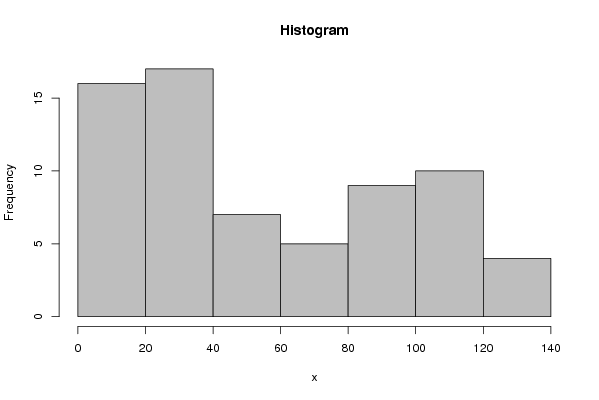## Free Statistics

of Irreproducible Research!

Author's title
Author*Unverified author*
R Software Modulerwasp_histogram.wasp
Title produced by softwareHistogram
Date of computationMon, 22 Feb 2010 13:20:51 -0700
Cite this page as followsStatistical Computations at FreeStatistics.org, Office for Research Development and Education, URL https://freestatistics.org/blog/index.php?v=date/2010/Feb/22/t12668704172m9vai3qx4rw9o9.htm/, Retrieved Tue, 07 Feb 2023 17:36:15 +0000
Statistical Computations at FreeStatistics.org, Office for Research Development and Education, URL https://freestatistics.org/blog/index.php?pk=73338, Retrieved Tue, 07 Feb 2023 17:36:15 +0000
QR Codes:Original text written by user:
IsPrivate?No (this computation is public)
User-defined keywordsKDGP1W22
Estimated Impact156
Family? (F = Feedback message, R = changed R code, M = changed R Module, P = changed Parameters, D = changed Data)
-     [Univariate Data Series] [historisch overzi...] [2010-02-09 19:37:30] [ebacbce6da0b74118b3de0fd7beb3336]
- RMPD    [Histogram] [] [2010-02-22 20:20:51] [ac302f869d0778eba7cafda3b14e71eb] [Current]
- R         [Histogram] [] [2010-05-19 13:20:28] [5a3034bcadf7735f2909fd8cdba599d5]
Feedback Forum

Post a new message
Dataseries X:
4.50
4.65
4.71
15.80
14.99
15.26
17.21
16.94
16.80
18.37
18.53
18.48
18.73
18.63
19.15
19.76
20.02
20.26
20.32
20.52
20.81
21.26
22.15
23.05
24.01
24.69
25.38
26.33
27.36
28.55
30.10
32.20
36.28
40.91
44.66
47.83
49.97
52.20
55.67
59.92
65.15
70.14
74.60
78.23
79.24
80.47
81.41
83.94
86.83
89.61
91.79
94.32
96.56
97.98
100.00
101.63
102.60
103.75
106.39
109.02
110.81
112.57
114.93
118.13
120.25
122.44
127.94
127.87


 Summary of computational transaction Raw Input view raw input (R code) Raw Output view raw output of R engine Computing time 1 seconds R Server 'Gwilym Jenkins' @ 72.249.127.135

\begin{tabular}{lllllllll}
\hline
Summary of computational transaction \tabularnewline
Raw Input & view raw input (R code)  \tabularnewline
Raw Output & view raw output of R engine  \tabularnewline
Computing time & 1 seconds \tabularnewline
R Server & 'Gwilym Jenkins' @ 72.249.127.135 \tabularnewline
\hline
\end{tabular}
%Source: https://freestatistics.org/blog/index.php?pk=73338&T=0

[TABLE]
[ROW][C]Summary of computational transaction[/C][/ROW]
[ROW][C]Raw Input[/C][C]view raw input (R code) [/C][/ROW]
[ROW][C]Raw Output[/C][C]view raw output of R engine [/C][/ROW]
[ROW][C]Computing time[/C][C]1 seconds[/C][/ROW]
[ROW][C]R Server[/C][C]'Gwilym Jenkins' @ 72.249.127.135[/C][/ROW]
[/TABLE]
Source: https://freestatistics.org/blog/index.php?pk=73338&T=0

Globally Unique Identifier (entire table): ba.freestatistics.org/blog/index.php?pk=73338&T=0

As an alternative you can also use a QR Code:

The GUIDs for individual cells are displayed in the table below:

 Summary of computational transaction Raw Input view raw input (R code) Raw Output view raw output of R engine Computing time 1 seconds R Server 'Gwilym Jenkins' @ 72.249.127.135

 Frequency Table (Histogram) Bins Midpoint Abs. Frequency Rel. Frequency Cumul. Rel. Freq. Density [0,20[ 10 16 0.235294 0.235294 0.011765 [20,40[ 30 17 0.25 0.485294 0.0125 [40,60[ 50 7 0.102941 0.588235 0.005147 [60,80[ 70 5 0.073529 0.661765 0.003676 [80,100[ 90 9 0.132353 0.794118 0.006618 [100,120[ 110 10 0.147059 0.941176 0.007353 [120,140] 130 4 0.058824 1 0.002941

\begin{tabular}{lllllllll}
\hline
Frequency Table (Histogram) \tabularnewline
Bins & Midpoint & Abs. Frequency & Rel. Frequency & Cumul. Rel. Freq. & Density \tabularnewline
[0,20[ & 10 & 16 & 0.235294 & 0.235294 & 0.011765 \tabularnewline
[20,40[ & 30 & 17 & 0.25 & 0.485294 & 0.0125 \tabularnewline
[40,60[ & 50 & 7 & 0.102941 & 0.588235 & 0.005147 \tabularnewline
[60,80[ & 70 & 5 & 0.073529 & 0.661765 & 0.003676 \tabularnewline
[80,100[ & 90 & 9 & 0.132353 & 0.794118 & 0.006618 \tabularnewline
[100,120[ & 110 & 10 & 0.147059 & 0.941176 & 0.007353 \tabularnewline
[120,140] & 130 & 4 & 0.058824 & 1 & 0.002941 \tabularnewline
\hline
\end{tabular}
%Source: https://freestatistics.org/blog/index.php?pk=73338&T=1

[TABLE]
[ROW][C]Frequency Table (Histogram)[/C][/ROW]
[ROW][C]Bins[/C][C]Midpoint[/C][C]Abs. Frequency[/C][C]Rel. Frequency[/C][C]Cumul. Rel. Freq.[/C][C]Density[/C][/ROW]
[ROW][C][0,20[[/C][C]10[/C][C]16[/C][C]0.235294[/C][C]0.235294[/C][C]0.011765[/C][/ROW]
[ROW][C][20,40[[/C][C]30[/C][C]17[/C][C]0.25[/C][C]0.485294[/C][C]0.0125[/C][/ROW]
[ROW][C][40,60[[/C][C]50[/C][C]7[/C][C]0.102941[/C][C]0.588235[/C][C]0.005147[/C][/ROW]
[ROW][C][60,80[[/C][C]70[/C][C]5[/C][C]0.073529[/C][C]0.661765[/C][C]0.003676[/C][/ROW]
[ROW][C][80,100[[/C][C]90[/C][C]9[/C][C]0.132353[/C][C]0.794118[/C][C]0.006618[/C][/ROW]
[ROW][C][100,120[[/C][C]110[/C][C]10[/C][C]0.147059[/C][C]0.941176[/C][C]0.007353[/C][/ROW]
[ROW][C][120,140][/C][C]130[/C][C]4[/C][C]0.058824[/C][C]1[/C][C]0.002941[/C][/ROW]
[/TABLE]
Source: https://freestatistics.org/blog/index.php?pk=73338&T=1

Globally Unique Identifier (entire table): ba.freestatistics.org/blog/index.php?pk=73338&T=1

As an alternative you can also use a QR Code:

The GUIDs for individual cells are displayed in the table below:

 Frequency Table (Histogram) Bins Midpoint Abs. Frequency Rel. Frequency Cumul. Rel. Freq. Density [0,20[ 10 16 0.235294 0.235294 0.011765 [20,40[ 30 17 0.25 0.485294 0.0125 [40,60[ 50 7 0.102941 0.588235 0.005147 [60,80[ 70 5 0.073529 0.661765 0.003676 [80,100[ 90 9 0.132353 0.794118 0.006618 [100,120[ 110 10 0.147059 0.941176 0.007353 [120,140] 130 4 0.058824 1 0.002941PNG link Postscript link PDF link

Parameters (Session):
par2 = grey ; par3 = FALSE ; par4 = Unknown ;
Parameters (R input):
par1 = ; par2 = grey ; par3 = FALSE ; par4 = Unknown ;
R code (references can be found in the software module):
par1 <- as.numeric(par1)if (par3 == 'TRUE') par3 <- TRUEif (par3 == 'FALSE') par3 <- FALSEif (par4 == 'Unknown') par1 <- as.numeric(par1)if (par4 == 'Interval/Ratio') par1 <- as.numeric(par1)if (par4 == '3-point Likert') par1 <- c(1:3 - 0.5, 3.5)if (par4 == '4-point Likert') par1 <- c(1:4 - 0.5, 4.5)if (par4 == '5-point Likert') par1 <- c(1:5 - 0.5, 5.5)if (par4 == '6-point Likert') par1 <- c(1:6 - 0.5, 6.5)if (par4 == '7-point Likert') par1 <- c(1:7 - 0.5, 7.5)if (par4 == '8-point Likert') par1 <- c(1:8 - 0.5, 8.5)if (par4 == '9-point Likert') par1 <- c(1:9 - 0.5, 9.5)if (par4 == '10-point Likert') par1 <- c(1:10 - 0.5, 10.5)bitmap(file='test1.png')if (is.na(par1)) {myhist<-hist(x,col=par2,main=main,xlab=xlab,right=par3)} else {if (par1 < 0) par1 <- 3if (par1 > 50) par1 <- 50myhist<-hist(x,breaks=par1,col=par2,main=main,xlab=xlab,right=par3)}dev.off()myhistn <- length(x)load(file='createtable')a<-table.start()a<-table.row.start(a)a<-table.element(a,hyperlink('histogram.htm','Frequency Table (Histogram)',''),6,TRUE)a<-table.row.end(a)a<-table.row.start(a)a<-table.element(a,'Bins',header=TRUE)a<-table.element(a,'Midpoint',header=TRUE)a<-table.element(a,'Abs. Frequency',header=TRUE)a<-table.element(a,'Rel. Frequency',header=TRUE)a<-table.element(a,'Cumul. Rel. Freq.',header=TRUE)a<-table.element(a,'Density',header=TRUE)a<-table.row.end(a)crf <- 0if (par3 == FALSE) mybracket <- '[' else mybracket <- ']'mynumrows <- (length(myhist$breaks)-1)for (i in 1:mynumrows) {a<-table.row.start(a)if (i == 1)dum <- paste('[',myhist$breaks[i],sep='')elsedum <- paste(mybracket,myhist$breaks[i],sep='')dum <- paste(dum,myhist$breaks[i+1],sep=',')if (i==mynumrows)dum <- paste(dum,']',sep='')elsedum <- paste(dum,mybracket,sep='')a<-table.element(a,dum,header=TRUE)a<-table.element(a,myhist$mids[i])a<-table.element(a,myhist$counts[i])rf <- myhist$counts[i]/ncrf <- crf + rfa<-table.element(a,round(rf,6))a<-table.element(a,round(crf,6))a<-table.element(a,round(myhist$density[i],6))a<-table.row.end(a)}a<-table.end(a)table.save(a,file='mytable.tab')